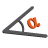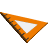You are here: Start » AVL.NET » Geometry 2D Angle Metrics

Geometry 2D Angle Metrics

IconNameDescriptionAngleBetweenDirectionsMeasures the rotation angle from first to second direction.AngleBetweenLinesMeasures the smaller and the larger angle between two lines.AngleBetweenSegmentsMeasures the angle between two segments with one of four possible metrics.AngleBetweenThreePointsMeasures the angle defined by three consecutive points.AngleBetweenVectorsMeasures the angle between two vectors.NormalizeAngleNormalizes an angle to the range <x, x+180) or <x, x+360).